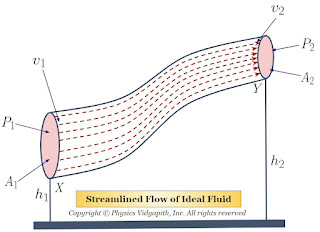## Nuclear Fuel Cycle

The nuclear fuel cycle refers to the series of steps involved in the production of nuclear power, from the mining of uranium ore to the disposal of nuclear waste.

Uranium Mining: The first step in the nuclear fuel cycle is the mining of uranium ore, which is typically extracted from underground mines or open-pit mines.

Uranium Enrichment: After the uranium ore is mined, it is transported to a facility where it is enriched, which involves increasing the proportion of uranium-235 isotopes in the uranium. This is done using centrifuges or other methods.

Fuel Fabrication: The enriched uranium is then processed into fuel pellets, which are loaded into fuel rods that will be used in nuclear reactors.

Nuclear Reactors: The fuel rods are then placed in a nuclear reactor, where they undergo a nuclear fission chain reaction that produces heat, which is used to generate electricity.

Spent Fuel Storage: After the fuel rods have been used in the reactor for several years, they become depleted and are considered "spent." They are removed from the reactor and stored in cooling pools at the nuclear power plant.

Reprocessing: In some countries, the spent fuel is then sent to a reprocessing facility, where the remaining uranium and plutonium in the fuel rods can be extracted and reused to make new fuel.

Disposal: Eventually, the spent fuel will need to be disposed of. In some countries, it is stored in long-term storage facilities, while in others, it is reprocessed or disposed of in deep geological repositories.

The nuclear fuel cycle is a complex process that requires careful management to ensure safety and minimize the risk of nuclear accidents or proliferation. Proper management and disposal of nuclear waste is a critical aspect of the nuclear fuel cycle, as it can remain radioactive for thousands of years and pose a hazard to human health and the environment.

## Popular Posts

### Bernoulli's Theorem and Derivation of Bernoulli's EquationStatement of Bernoulli's Theorem: When an ideal fluid (i.e incompressible and non-viscous Liquid or Gas) flows in streamlined motion from one place to another, then the total energy per unit volume (i.e Pressure energy + Kinetic Energy + Potential Energy) at each and every of its path is constant. $P+\frac{1}{2}\rho v^{2} + \rho gh= constant$ Derivation of Bernoulli's Theorem Equation: Let us consider that an incompressible and non-viscous liquid is flowing in streamlined motion through a tube $XY$ of the non-uniform cross-section. Now Consider: The Area of cross-section $X$ = $A_{1}$ The Area of cross-section $Y$ = $A_{2}$ The velocity per second (i.e. equal to distance) of fluid at cross-section $X$ = $v_{1}$ The velocity per second (i.e. equal to distance) of fluid at cross-section $Y$ = $v_{2}$ The Pressure of fluid at cross-section $X$ = $P_{1}$ The Pressure of fluid at cross-section $Y$ = $P_{2}$ The height of cross-section $X$ from